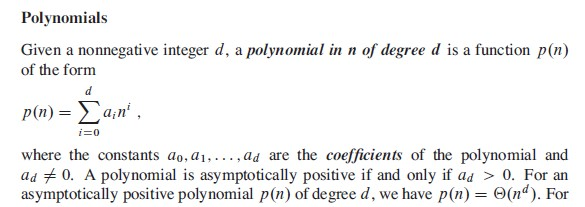Asymptotic behavior of polynomials

Let

$p(n) = \sum_{i = 0}^d a_in^i$

where $$a_d > 0$$, be a degree-$$d$$ polynomial in $$n$$, and let $$k$$ be a constant. Use the definitions of the asymptotic notations to prove the following properties.

1. If $$k \ge d$$, then $$p(n) = O(n^k)$$.
2. If $$k \le d$$, then $$p(n) = \Omega(n^k)$$.
3. If $$k = d$$, then $$p(n) = \Theta(n^k)$$.
4. If $$k > d$$, then $$p(n) = o(n^k)$$.
5. If $$k < d$$, then $$p(n) = \omega(n^k)$$.

Before jumping on to the proofs, let’s revisit the polynomials related notes from section 3.2 in the book:And this is exactly what we have here. An asymptotically positive polynomial! Which makes c, i.e. when $$k = d$$, $$p(n)= \Theta(n^k)$$, a trivial proof. And others can also be derived from the same.

However, let’s assume the goal of this exercise is to prove the claim made in the book.

We can prove each of these either with analytical logic or mathematical or derivation or using even more rigorous mathematics. I’ll limit myself to the first two category and leave some reference for the third one at the end of the solution.

#### C. Theta or Asymptotic Bound

Let’s try to prove c first as others can be followed from that.

##### Analytical Approach

The largest term in the polynomial is $$a_dn^d$$, so the polynomial cannot grow neither slower nor faster than $$n^d$$. Hence, $$p(n) = \Theta(n^k)$$.

##### Mathematical Approach

The polynomial can be written as:

\begin{aligned} p(n) &= \sum_{i = 0}^d a_in^i \\ &= a_dn^d + \sum_{i = 0}^{d - 1} a_in^i \\ &= a_dn^d + n^d \sum_{i = 0}^{d - 1} a_in^{i -d} \\ &= a_dn^d + n^d Q_n \\ &= n^d(a_d + Q_n) \end{aligned}

Where, $$Q_n = \sum_{i = 0}^{d - 1} a_in^{i -d}$$

Now note that $$Q_n$$ is the sum of powers of $$n$$ multiplied by some constants, and the powers of $$n$$ are all less than or equal to -1. This follows from the fact that $$(i - d) \leq -1$$. Now for sufficiently large $$n$$, $$Q_n$$ approaches zero. And that in turn means, before it reaches zero, we can find a positive integer $$n_0$$, such that: $$\vert Q_n \vert \leq 0.5a_d$$ for all $$n \geq n_0$$.

Following from that,

$-0.5a_d \leq Q_n \leq 0.5a_d \\[1ex] a_d - 0.5a_d \leq a_d + Q_n \leq a_d + 0.5a_d \\[1ex] n^d (a_d - 0.5a_d) \leq n^d (a_d + Q_n) \leq n^d (a_d + 0.5a_d) \\[1ex] 0.5a_d \cdot n^d \leq n^d (a_d + Q_n) \leq 1.5a_d \cdot n^d \\[1ex] 0.5a_d \cdot n^d \leq p(n) \leq 1.5a_d \cdot n^d$

So, if we pick $$c_1 = 0.5a_d$$ and $$c_2 = 1.5a_d$$, we have $$c_1n^d \leq p(n) \leq c_2n^d$$.

In other words, $$p(n) = \Theta(n^d)$$.

And when $$k = d$$, it means $$p(n) = \Theta(n^k)$$.

#### A. Asymptotic Upper Bound

Analytically. as $$k \geq d$$, asymptotically $$n^k$$ grows faster or at same rate than $$n^d$$ for sufficiently large $$n$$. Hence, $$p(n) = O(n^k)$$.

Mathematically, from c we can write:

$0 \leq p(n) \leq 1.5a_d \cdot n^d \leq 1.5a_d \cdot n^k$

So, if we pick $$c_1 = 1.5a_d$$, we have $$0 \leq p(n) \leq c_1n^k$$.

In other words, $$p(n) = O(n^k)$$.

#### B. Asymptotic Lower Bound

Analytically. as $$k \leq d$$, asymptotically $$n^k$$ grows slower or at same rate than $$n^d$$ for sufficiently large $$n$$. Hence, $$p(n) = \Omega(n^k)$$.

Mathematically, from c we can write:

$0 \leq 0.5a_d \cdot n^k \leq 0.5a_d \cdot n^d \leq p(n)$

So, if we pick $$c_1 = 0.5a_d$$, we have $$0 \leq c_1n^k \leq p(n)$$.

In other words, $$p(n) = \Omega(n^k)$$.

#### D. Asymptotic Non-tight Upper Bound

We can easily prove this by removing the equality from the proof of a.

Other than that we can use the limit definition of $$o$$-notation:

\begin{aligned} \lim_{n \to \infty} \frac {p(n)} {n^k} & = \lim_{n \to \infty} \frac {n^d(a_d + Q_n)} {n^k} \\ & = \lim_{n \to \infty} \frac {a_dn^d} {n^k} \\ & = \lim_{n \to \infty} \frac {a_d} {n^{k - d}} \\ & = 0 \end {aligned}

Hence, $$p(n) = o(n)$$.

#### E. Asymptotic Non-tight Lower Bound

We can easily prove this by removing the equality from the proof of b.

Other than that we can use the limit definition of $$\omega$$-notation:

\begin{aligned} \lim_{n \to \infty} \frac {p(n)} {n^k} & = \lim_{n \to \infty} \frac {n^d(a_d + Q_n)} {n^k} \\ & = \lim_{n \to \infty} \frac {a_dn^d} {n^k} \\ & = \lim_{n \to \infty} a_d n^{d - k} \\ & = \infty \end {aligned}

Hence, $$p(n) = \omega(n)$$.